Anúncio

# Gases

21 de Sep de 2017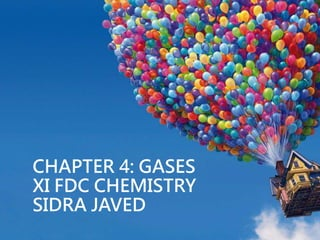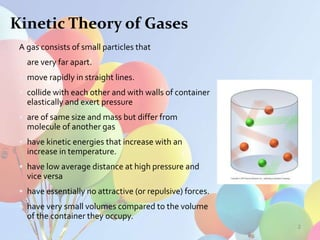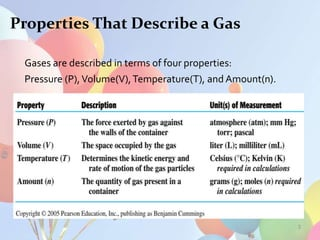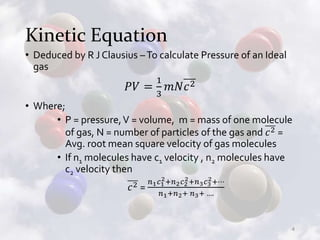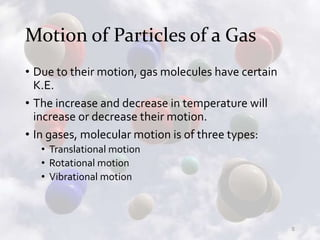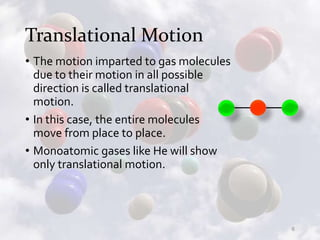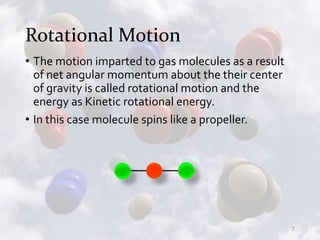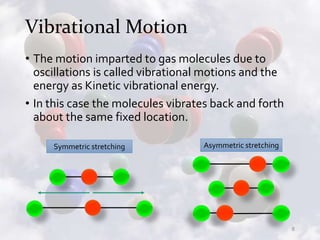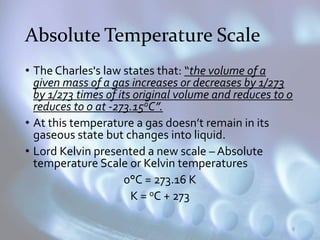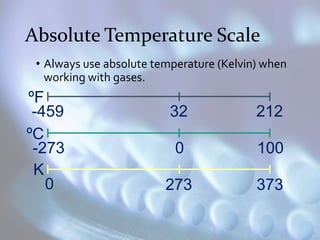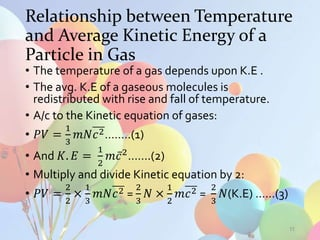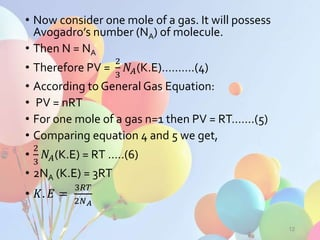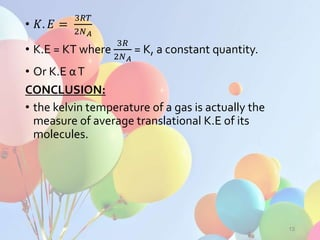1 de 14
Anúncio

### Gases

1. 1 CHAPTER 4: GASES XI FDC CHEMISTRY SIDRA JAVED
2. Kinetic Theory of Gases A gas consists of small particles that • are very far apart. • move rapidly in straight lines. • collide with each other and with walls of container elastically and exert pressure • are of same size and mass but differ from molecule of another gas • have kinetic energies that increase with an increase in temperature. • have low average distance at high pressure and vice versa • have essentially no attractive (or repulsive) forces. • have very small volumes compared to the volume of the container they occupy. 2
3. Properties That Describe a Gas Gases are described in terms of four properties: Pressure (P),Volume(V),Temperature(T), and Amount(n). 3
4. Kinetic Equation • Deduced by R J Clausius –To calculate Pressure of an Ideal gas 𝑃𝑉 = 1 3 𝑚𝑁𝑐2 • Where; • P = pressure,V = volume, m = mass of one molecule of gas, N = number of particles of the gas and 𝑐2 = Avg. root mean square velocity of gas molecules • If n1 molecules have c1 velocity , n2 molecules have c2 velocity then 𝑐2 = 𝑛1 𝑐1 2+𝑛2 𝑐2 2+𝑛3 𝑐3 2+⋯ 𝑛1+𝑛2+ 𝑛3+ …. 4
5. Motion of Particles of a Gas • Due to their motion, gas molecules have certain K.E. • The increase and decrease in temperature will increase or decrease their motion. • In gases, molecular motion is of three types: • Translational motion • Rotational motion • Vibrational motion 5
6. Translational Motion • The motion imparted to gas molecules due to their motion in all possible direction is called translational motion. • In this case, the entire molecules move from place to place. • Monoatomic gases like He will show only translational motion. 6
7. Rotational Motion • The motion imparted to gas molecules as a result of net angular momentum about the their center of gravity is called rotational motion and the energy as Kinetic rotational energy. • In this case molecule spins like a propeller. 7
8. Vibrational Motion • The motion imparted to gas molecules due to oscillations is called vibrational motions and the energy as Kinetic vibrational energy. • In this case the molecules vibrates back and forth about the same fixed location. 8 Symmetric stretching Asymmetric stretching
9. Absolute Temperature Scale • The Charles's law states that: “the volume of a given mass of a gas increases or decreases by 1/273 by 1/273 times of its original volume and reduces to 0 reduces to 0 at -273.15oC”. • At this temperature a gas doesn’t remain in its gaseous state but changes into liquid. • Lord Kelvin presented a new scale – Absolute temperature Scale or Kelvin temperatures 0°C = 273.16 K K = oC + 273 9
10. Absolute Temperature Scale • Always use absolute temperature (Kelvin) when working with gases. ºF ºC K -459 32 212 -273 0 100 0 273 373
11. Relationship between Temperature and Average Kinetic Energy of a Particle in Gas • The temperature of a gas depends upon K.E . • The avg. K.E of a gaseous molecules is redistributed with rise and fall of temperature. • A/c to the Kinetic equation of gases: • 𝑃𝑉 = 1 3 𝑚𝑁𝑐2……..(1) • And 𝐾. 𝐸 = 1 2 𝑚 𝑐2 …….(2) • Multiply and divide Kinetic equation by 2: • 𝑃𝑉 = 2 2 × 1 3 𝑚𝑁𝑐2 = 2 3 𝑁 × 1 2 𝑚𝑐2 = 2 3 𝑁(K.E) ……(3) 11
12. • Now consider one mole of a gas. It will possess Avogadro’s number (NA) of molecule. • Then N = NA • Therefore PV = 2 3 𝑁𝐴(K.E)……….(4) • According to General Gas Equation: • PV = nRT • For one mole of a gas n=1 then PV = RT…….(5) • Comparing equation 4 and 5 we get, • 2 3 𝑁𝐴(K.E) = RT …..(6) • 2NA (K.E) = 3RT • 𝐾. 𝐸 = 3𝑅𝑇 2𝑁 𝐴 12
13. • 𝐾. 𝐸 = 3𝑅𝑇 2𝑁 𝐴 • K.E = KT where 3𝑅 2𝑁 𝐴 = K, a constant quantity. • Or K.E αT CONCLUSION: • the kelvin temperature of a gas is actually the measure of average translational K.E of its molecules. 13
14. End of Lesson 1 sidra.javedali@gmail.com 14
Anúncio